# Difference between revisions of "Sine-cubed function"

This article is about a particular function from a subset of the real numbers to the real numbers. Information about the function, including its domain, range, and key data relating to graphing, differentiation, and integration, is presented in the article.
View a complete list of particular functions on this wiki
For functions involving angles (trigonometric functions, inverse trigonometric functions, etc.) we follow the convention that all angles are measured in radians. Thus, for instance, the angle of$90\,^\circ$ is measured as$\pi/2$.

## Definition

This function, denoted$\sin^3$, is defined as the composite of the cube function and the sine function. Explicitly, it is the map: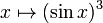$\! x \mapsto (\sin x)^3$

For brevity, we write$(\sin x)^3$ or$\sin^3x$.

## Key data

Item Value
Default domain all real numbers, i.e., all of$\R$
range the closed interval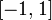$[-1,1]$, i.e.,$\{ y \mid -1 \le y \le 1 \}$
absolute maximum value: 1, absolute minimum value: -1
period$2\pi$, i.e.,$360\,^\circ$
local maximum values and points of attainment All local maximum values are equal to 1, and they are attained at all points of the form$2n\pi + \pi/2$ where$n$ varies over integers.
local minimum values and points of attainment All local minimum values are equal to -1, and they are attained at all points of the form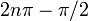$2n\pi - \pi/2$ where$n$ varies over integers.
points of inflection (both coordinates) All points of the form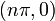$(n\pi,0)$, as well as points of the form$(n\pi + \alpha, (-1)^n2\sqrt{2}/3\sqrt{3})$ and$(n\pi + \pi - \alpha, (-1)^n2\sqrt{2}/3\sqrt{3})$ where$\alpha = \arcsin(\sqrt{2/3})$ where$n$ varies over integers.
derivative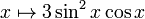$x \mapsto 3\sin^2x \cos x$
second derivative$x \mapsto 6 \sin x \cos^2x - 3\sin^3x = 6 \sin x - 9 \sin^3x$
antiderivative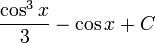$\frac{\cos^3x}{3} - \cos x + C$
important symmetries odd function (follows from composite of odd functions is odd, and the fact that the cube function and sine function are both odd)
half turn symmetry about all points of the form$(n\pi,0)$
mirror symmetry about all lines$x = n\pi + \pi/2$.

## Identities

We have the identity:$\! \sin^3 x = \frac{3\sin x - \sin(3x)}{4}$

## Graph

Here is the basic graph, drawn on the interval$[-2\pi,2\pi]$:

Here is a more close-up graph, drawn on the interval$[-\pi,\pi]$. The thick black dots correspond to local extreme values, and the thick red dots correspond to points of inflection.

## Differentiation

### First derivative

To differentiate once, we use the chain rule for differentiation. Explicitly, we consider the function as the composite of the cube function and the sine function, so the cube function is the outer function and the sine function is the inner function.

We get:$\frac{d}{dx}[(\sin x)^3] = 3(\sin x)^2 \cos x = 3\sin^2x \cos x$

## Integration

### First antiderivative: standard method

We rewrite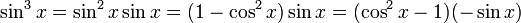$\sin^3x = \sin^2x \sin x = (1 - \cos^2x)\sin x = (\cos^2x - 1)(-\sin x)$ and then do integration by u-substitution where$u = \cos x$. Explicitly:$\int \sin^3 x \, dx = \int \sin^2x \sin x \, dx = \int (1 - \cos^2x) \sin x \, dx = \int (\cos^2x - 1) (-\sin x) \, dx$

Now put$u = \cos x$. We have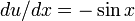$du/dx = - \sin x$, so we can replace$(-\sin x) \, dx$ by$du$, and we get: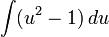$\int (u^2 - 1) \, du$

By polynomial integration, we get: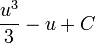$\frac{u^3}{3} - u + C$

Plugging back$u = \cos x$, we get: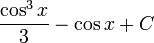$\! \frac{\cos^3x}{3} - \cos x + C$.

Here,$C$ is an arbitrary real constant.

### First antiderivative: using triple angle formula

An alternate method for integrating the function$\sin^3 x$ is to use the identity:$\! \sin^3x = \frac{3\sin x - \sin(3x)}{4}$

We thus get: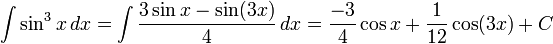$\! \int \sin^3x \, dx = \int \frac{3\sin x - \sin(3x)}{4} \, dx = \frac{-3}{4} \cos x + \frac{1}{12} \cos(3x) + C$

This answer looks superficially different from the other answer. However, using the identity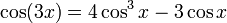$\cos(3x) = 4\cos^3x - 3\cos x$, we can verify that the antiderivatives are exactly the same.

### Repeated antidifferentiation

The antiderivative of$\sin^3$ involves cos^3 and cos, both of which can be antidifferentiated, and this now involves sin^3 and sin. We can thus antidifferentiate (i.e., integrate) the function any number of times, with the antiderivative expression alternating between a cubic function of sine and a cubic function of cosine.

## Power series and Taylor series

### Computation of power series

We can use the identity:$\! \sin^3x = \frac{3\sin x - \sin(3x)}{4}$

We have the power series:$\! \sin x = x - \frac{x^3}{3!} + \frac{x^5}{5!} - \dots = \sum_{k=0}^\infty \frac{(-1)^kx^{2k+1}}{(2k+1)!}$

We thus get the power series:$\! \sin(3x) = (3x) - \frac{(3x)^3}{3!} + \frac{(3x)^5}{5!} - \dots = \sum_{k=0}^\infty \frac{(-1)^k3^{2k+1}x^{2k+1}}{(2k+1)!}$

Plugging into the formula, we get:$\! \sin^3x = \sum_{k=0}^\infty \frac{(-1)^k(3 - 3^{2k+1})}{4 \cdot (2k+1)!}$

The first few terms are as follows: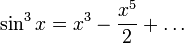$\! \sin^3x = x^3 - \frac{x^5}{2} + \dots$